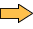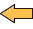Main Menu: Home |  News |  Manual |  Object Reference |  Crypto 101 |  Download & Buy |  Live Demo |  Support |  Contact Navigator: Home |  Manual |  Chapter 3: One-way Hash FunctionsChapter 10: Microsoft .NET and AspEncrypt Compatibility
10.1 Introduction
10.3 ImportRawKey: Byte Order Reversal
10.4 MD5 Hash & 3DES Keys
10.5 Encoding.Unicode vs. Encoding.UTF8

When converting legacy ASP applications that use AspEncrypt to .NET, developers often run into various key compatibility issues that prevent data encrypted with AspEncrypt from being correctly decrypted with the .NET cryptography objects, or vice versa. This chapter is dedicated to addressing many of these issues and providing code samples in classic ASP and .NET demonstrating how to create cipher keys that are compatible between the two platforms.

The cipher key compatibility issues between CryptoAPI-based AspEncrypt and the .NET framework may arise for the following reasons:

• Symmetric encryption in AspEncrypt is usually performed with the help of the GenerateKeyFromPassword method, but there is no direct equivalent for this method in .NET.
• CryptoAPI/AspEncrypt handle 40-bit RC2 key generation differently than .NET.
• CryptoAPI/AspEncrypt and .NET store the key bytes in the opposite orders.
• CryptoAPI/AspEncrypt and .NET use different algorithms for key padding in case the hash function produces a shorter value than necessary for the cipher key (such as, MD5 and 3DES.)
• When converting a text password to an encryption key, using different character encodings produces different hash values and, therefore, incompatible keys.

These and other issues will be covered in detail below.

10.2.1 Standard Key Length Handling

AspEncrypt's CryptoContext.GenerateKeyFromPassword method takes 4 arguments, of which only the first one, the password string, is required. The other three arguments are the hash algorithm (SHA by default), cipher algorithm (RC2 by default) and key length (128 by default for the Enhanced, Strong cryptographic providers, and 40 for the Base provider.)

Consider the following code snippet:

VBScript
```Set CM = Server.CreateObject("Persits.CryptoManager")
Set Context = CM.OpenContext("", True)
Set Blob = Key.EncryptText("Hello World!")
Response.Write Blob.Base64```

Assuming the Strong or Enhanced cryptographic provider is used, this code snippet produces the following output:

16Ij1qo4gRbfXuaEE3uTtQ==

The snippet above implicitly uses the SHA hash function to convert the specified text string to the key bits, and produces a 128-bit RC2 cipher key. The 160-bit hash function generates more data than necessary for the key, so the rest of the bits is discarded.

The .NET equivalent of the code above is as follows:
C#
```RC2CryptoServiceProvider rc2 = new RC2CryptoServiceProvider();
SHA1CryptoServiceProvider sha = new SHA1CryptoServiceProvider();
byte[] keybytes = new byte;
Array.Copy(shahash, keybytes, 16);

rc2.Key = keybytes;
rc2.IV = new byte[rc2.BlockSize / 8];

ICryptoTransform ctr = rc2.CreateEncryptor();

byte[] plaintext = Encoding.UTF8.GetBytes("Hello World!");
byte[] ciphertext = ctr.TransformFinalBlock(plaintext, 0, (int)plaintext.Length);
Response.Write( Convert.ToBase64String(ciphertext) );```

The output is also 16Ij1qo4gRbfXuaEE3uTtQ==.

Note that the .NET code requires that an initialization vector be specified even if it is all 0s. Omitting the .IV property results in random output.

10.2.2 Short (40-bit) Key Handling

40-bit encryption is extremely weak and should never be used. However, some very old legacy systems originally designed for Windows NT and Windows 2000 still use 40-bit RC2 and RC4 keys. Consider the following ASP code:

VBScript
```Set CM = Server.CreateObject("Persits.CryptoManager")
Set Context = CM.OpenContext("", True)
Response.Write Key.EncryptText("Hello World!").Base64```

Output: 3PmOk7WfLPRlYxa+PTboYA==

To generate a compatible in .NET code, it is not sufficient to change the C# code snippet above by replacing the two lines

byte[] keybytes = new byte;
Array.Copy(shahash, keybytes, 16);
' 128-bit

with

byte[] keybytes = new byte;
Array.Copy(shahash, keybytes, 5);
' 40-bit

The matching .NET code must set the UseSalt property to true, as follows:

C#
```RC2CryptoServiceProvider rc2 = new RC2CryptoServiceProvider();
SHA1CryptoServiceProvider sha = new SHA1CryptoServiceProvider();
byte[] keybytes = new byte;
Array.Copy(shahash, keybytes, 5);
rc2.Key = keybytes;
rc2.IV = new byte[rc2.BlockSize / 8];
rc2.UseSalt = true;
ICryptoTransform ctr = rc2.CreateEncryptor();

byte[] plaintext = Encoding.UTF8.GetBytes("Hello World!");
byte[] ciphertext = ctr.TransformFinalBlock(plaintext, 0, (int)plaintext.Length);
Response.Write( Convert.ToBase64String(ciphertext) );```

Output: 3PmOk7WfLPRlYxa+PTboYA==

10.3 ImportRawKey: Byte Order Reversal
When the encryption key is not derived from a password but specified in the form of a raw bit sequence (usually Hex- or Base64-encoded), the AspEncrypt method CryptoContext.ImportRawKey should be used. Since the .NET framework stores its key bytes in the Big-endian order and CryptoAPI/AspEncrypt in the Little-endian order, the third Booleam argument to ImportRawKey (ReverseBytes) should be set to True.

Consider the following .NET code that encrypts the string "Hello World!" with a specified 256-bit AES key and 128-bit initialization vector. The key and IV are specified as follows (Hex format):

Key: DC60F98E93B59F2CF4656316BE3D36E8ACB804C340EF95A24C3BCB6DD75F976F
IV: 950F6F4F3C018ED60F98C40AFD6D70E5

C#
```static byte[] HexToBytes(string hexString)
{
byte[] Hex = new byte[hexString.Length / 2];
for (int index = 0; index < Hex.Length; index++)
{
string byteValue = hexString.Substring(index * 2, 2);
Hex[index] = byte.Parse(byteValue, NumberStyles.HexNumber, CultureInfo.InvariantCulture);
}

return Hex;
}

void AESEncrypt()
{
Rijndael Aes = Rijndael.Create();
Aes.Key = HexToBytes("DC60F98E93B59F2CF4656316BE3D36E8ACB804C340EF95A24C3BCB6DD75F976F");
Aes.IV = HexToBytes("950F6F4F3C018ED60F98C40AFD6D70E5");

ICryptoTransform ctr = Aes.CreateEncryptor(Aes.Key, Aes.IV);
byte[] plaintext = Encoding.UTF8.GetBytes("Hello World!");
Message.Text = Convert.ToBase64String(ctr.TransformFinalBlock(plaintext, 0, (int)plaintext.Length));
}```

Output: c11uHEL6u/vVHi8Oh8iQXg==

The matching AspEncrypt-based script calls ImportRawKey with the 3d argument set to True, as follows:

VBScript
```Set CM = Server.CreateObject("Persits.CryptoManager")
Set Context = CM.OpenContextEx("Microsoft Enhanced RSA and AES Cryptographic Provider", "", True)

Set KeyBlob = CM.CreateBlob
KeyBlob.Hex = "DC60F98E93B59F2CF4656316BE3D36E8ACB804C340EF95A24C3BCB6DD75F976F"

Set IVBlob = CM.CreateBlob
IVBlob.Hex = "950F6F4F3C018ED60F98C40AFD6D70E5"

Set Key = Context.ImportRawKey(KeyBlob, calgAES256, True)
Key.SetIV IVBlob

Encoded.Text = Key.EncryptText("Hello World!").Base64```

Output: c11uHEL6u/vVHi8Oh8iQXg==

10.4 MD5 Hash & 3DES Keys
The 128-bit MD5 hash function has been cracked and is considered obsolete and unsuitable for digital signing. However it is still widely used for key generation.

Some legacy systems use Triple-DES keys derived from passwords using the MD5 hash, which causes a compatibility problem as Triple-DES uses 24-byte keys while MD5 only provides 16 bytes of key data. CryptoAPI/AspEncrypt uses one algorithm to convert 16-bit data to a 24-bit key, while .NET uses another.

(Note: Triple-DES is a 168-bit cipher but its keys are always specified as 24-byte (192-bit) sequences. The last bit of each byte is ignored.)

Consider the following AspEncrypt-based code which uses an MD5-derived password to encrypt the string "Hello World!":

VBScript
```Set CM = Server.CreateObject("Persits.CryptoManager")
Set Context = cm.OpenContext("", True)

Response.Write Key.EncryptText("Hello World!").Base64```

Output: pwmDLnkeQVueCLcltuvahQ==

1. Form a 64-byte buffer by repeating the constant 0x36 64 times. Let k be the length of the hash value that is represented by the input parameter hBaseData. Set the first k bytes of the buffer to the result of an XOR operation of the first k bytes of the buffer with the hash value that is represented by the input parameter hBaseData.
2. Form a 64-byte buffer by repeating the constant 0x5C 64 times. Set the first k bytes of the buffer to the result of an XOR operation of the first k bytes of the buffer with the hash value that is represented by the input parameter hBaseData.
3. Hash the result of step 1 by using the same hash algorithm as that used to compute the hash value that is represented by the hBaseData parameter.
4. Hash the result of step 2 by using the same hash algorithm as that used to compute the hash value that is represented by the hBaseData parameter.
5. Concatenate the result of step 3 with the result of step 4.
6. Use the first n bytes of the result of step 5 as the derived key.

The .NET code that implements this algorithm is as follows:
C#
```byte[] ShortKeyToLongKey(byte[] hash, int nSize)
{
byte[] output = new byte[nSize];

byte[] buffer = new byte;
for (int i = 0; i < 64; i++)
buffer[i] = 0x36;

int k = hash.Length;
for (int i = 0; i < k; i++)
buffer[i] ^= hash[i];

byte[] buffer2 = new byte;
for (int i = 0; i < 64; i++)
buffer2[i] = 0x5C;

for (int i = 0; i < k; i++)
buffer2[i] ^= hash[i];

MD5 md5 = new MD5CryptoServiceProvider();

byte[] hash1 = md5.ComputeHash(buffer);
byte[] hash2 = md5.ComputeHash(buffer2);

int m = 0;
for (m = 0; m < hash1.Length; m++)
output[m] = hash1[m];

for (m = 0; m < hash2.Length; m++)
{
if (m + hash1.Length >= output.Length)
break;

output[m + hash1.Length] = hash2[m];
}

return output;
}

void Encrypt3DES()
{
TripleDESCryptoServiceProvider tdes = new TripleDESCryptoServiceProvider();
MD5CryptoServiceProvider md5 = new MD5CryptoServiceProvider();

byte[] keybytes = ShortKeyToLongKey(md5hash, 24);
tdes.Key = keybytes;
tdes.IV = new byte[tdes.BlockSize / 8];

ICryptoTransform ctr = tdes.CreateEncryptor(tdes.Key, tdes.IV);
byte[] plaintext = Encoding.UTF8.GetBytes("Hello World!");
Response.Write( Convert.ToBase64String(ctr.TransformFinalBlock(plaintext, 0, (int)plaintext.Length)) );
}
```

Output: pwmDLnkeQVueCLcltuvahQ==

Let us now consider the opposite scenario: we are given a .NET script that plugs a MD5-derived 128-bit sequence directly into the TirpleDES's Key property without any further processing:

C#
```TripleDESCryptoServiceProvider tdes = new TripleDESCryptoServiceProvider();
MD5CryptoServiceProvider md5 = new MD5CryptoServiceProvider();

tdes.Key = md5hash;
tdes.IV = new byte[tdes.BlockSize / 8];

ICryptoTransform ctr = tdes.CreateEncryptor(tdes.Key, tdes.IV);
byte[] plaintext = Encoding.UTF8.GetBytes("Hello World!");
Response.Write( Convert.ToBase64String(ctr.TransformFinalBlock(plaintext, 0, (int)plaintext.Length)) );
}
```

Output: FgXZCTzxqg9klWpMciy7cw==

The .NET framework uses a much simpler algorithm to convert a 16-byte sequence into 24 bytes: it simply concatenates the given 16 bytes of data with the first 8 bytes of the same data to form a 24-byte sequence. For example, if the input 16-byte data is 000102030405060708090A0B0C0D0E0F, the resultant 24-byte data is 000102030405060708090A0B0C0D0E0F0001020304050607.

The following AspEncrypt-based code implements this algorithm:

VBScript
```Set CM = Server.CreateObject("Persits.CryptoManager")
Set Context = CM.OpenContext("", True)
Set hash = Context.CreateHash(calgMD5)
HexKey = hash.Value.Hex
HexKey = HexKey & Left(HexKey, 8 * 2)

Set KeyBlob = CM.CreateBlob
KeyBlob.Hex = HexKey

Set Key = Context.ImportRawKey(KeyBlob, calg3DES, True)
Response.Write Key.EncryptText("Hello World!").Base64 ```

Output: FgXZCTzxqg9klWpMciy7cw==

10.5 Encoding.Unicode vs. Encoding.UTF8
The AspEncrypt methods CryptoKey.EncryptText and CryptoHash.AddText internally use the UTF-8 encoding to convert the Unicode text arguments to a sequence of bytes. This corresponds to .NET's Encoding.UTF8.GetBytes method.

For example, the following AspEncrypt-based code snippet computes the hash of a UTF-8 encoded text string:

VBScript
```Set CM = Server.CreateObject("Persits.CryptoManager")
Set Context = CM.OpenContext("", True)
Set Hash = Context.CreateHash(calgSHA)
Response.Write Hash.Value.Base64```

Output: 1c1m8Gyxk1xiFKx0MQzNzkW9kWA=

The .NET equivalent is:

C#
```SHA1CryptoServiceProvider sha = new SHA1CryptoServiceProvider();
byte [] byteseq = sha.ComputeHash(Encoding.UTF8.GetBytes("My password"));
Message.Text = Convert.ToBase64String(byteseq);```

Output: 1c1m8Gyxk1xiFKx0MQzNzkW9kWA=

However, if the .NET code uses the Encoding.Unicode encoding instead of Encoding.UTF8, the matching AspEncrypt methods to use would be CryptoKey.EncryptTextWide and CryptoHash.AddTextWide.

VBScript
```Set CM = Server.CreateObject("Persits.CryptoManager")
Set Context = CM.OpenContext("", True)
Set Hash = Context.CreateHash(calgSHA)
Response.Write Hash.Value.Base64```

Output: 1mtjUQkRFeImD3lD7r7Dy+IDtoE=

The .NET equivalent is:

C#
```SHA1CryptoServiceProvider sha = new SHA1CryptoServiceProvider();
byte [] byteseq = sha.ComputeHash(Encoding.Unicode.GetBytes("My password"));
Message.Text = Convert.ToBase64String(byteseq);```

Output: 1mtjUQkRFeImD3lD7r7Dy+IDtoE=